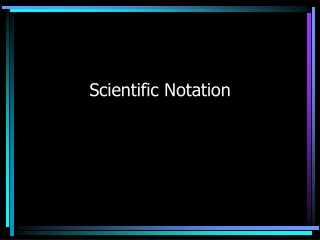Download PresentationScientific Notation

# Scientific Notation

Download Presentation## Scientific Notation

- - - - - - - - - - - - - - - - - - - - - - - - - - - E N D - - - - - - - - - - - - - - - - - - - - - - - - - - -
##### Presentation Transcript

1. Scientific Notation

2. When using Scientific Notation, there are two kinds of exponents: positive and negative Positive Exponent: 2.35 x 108 Negative Exponent: 3.97 x 10-7

3. When changing scientific notation to standard notation, the exponent tells you if you should move the decimal: With a positiveexponent, move the decimal to the right: 4.08 x 103 = 4 0 8 Don’t forget to fill in your zeroes!

4. When changing scientific notation to standard notation, the exponent tells you if you should move the decimal: With a negative exponent, move the decimal to the left: 4.08 x 10-3 = 4 0 8 Don’t forget to fill in your zeroes!

5. An easy way to remember this is: • If an exponent is positive, the number gets larger, so move the decimal to the right. • If an exponent is negative, the number gets smaller, so move the decimal to the left.

6. The exponent also tells how many spaces to move the decimal: 4.08 x 103 = 4 0 8 In this problem, the exponent is +3, so the decimal moves 3 spaces to the right.

7. The exponent also tells how many spaces to move the decimal: 4.08 x 10-3 = 4 0 8 In this problem, the exponent is -3, so the decimal moves 3 spaces to the left.

8. Try changing these numbers from Scientific Notation to Standard Notation: • 9.678 x 104 • 7.4521 x 10-3 • 8.513904567 x 107 • 4.09748 x 10-5 96780 .0074521 85139045.67 .0000409748

9. When changing from Standard Notation to Scientific Notation: 1) First, move the decimal after the first whole number: 3 2 5 8 2) Second, add your multiplication sign and your base (10). 3 . 2 5 8 x 10 3) Count how many spaces the decimal moved and this is the exponent. 3 . 2 5 8 x 10 3 3 2 1

10. When changing from Standard Notation to Scientific Notation: 4) See if the original number is greaterthan or less than one. • If the number is greater than one, the exponent will be positive. 348943 = 3.48943 x 105 • If the number is less than one, the exponent will be negative. .0000000672 = 6.72 x 10-8

11. Try changing these numbers from Standard Notation to Scientific Notation: • 9872432 • .0000345 • .08376 • 5673 9.872432 x 106 3.45 x 10-5 8.376 x 10-2 5.673 x 103